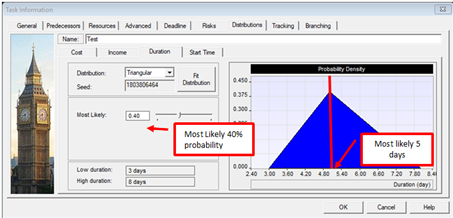Page 1 of 1

### Modifying most likely values in the Task Information > Distribution dialog box.

Posted: Thu Mar 14, 2019 1:49 pm
How does changing the Most Likely value for Triangular distributions affect simulation results?

### Re: Modifying most likely values in the Task Information > Distribution dialog box.

Posted: Thu Mar 14, 2019 1:52 pm
When you assign a statistical distribution to an activity that has best case, most likely, and worst case estimates, the software calculates the probability distribution function based on the estimates and the statistical distribution type. The Distribution tab in the Task Information dialog box allows some “fine tuning” of this probability distribution function by modifying available parameters. For triangular distribution, this parameter is the most likely.
If you change the Most Likely, which is the base estimate, it will skew the distribution which will affect the simulation results. For example, if you have an activity with estimates for best case, most likely, and worst case of 3,5, and 8 days respectively, the calculated probability of the most likely is 40%.If you modify the most likely probability, it will change the acutal most likely value used in the simulation and skew the results accordingly. These new parameters will update the Most Likely estimate entered in the Gantt view.### Re: Modifying most likely values in the Task Information > Distribution dialog box.

Posted: Thu Mar 14, 2019 1:55 pm
What do the different options for Normal distributions mean?

### Re: Modifying most likely values in the Task Information > Distribution dialog box.

Posted: Thu Mar 14, 2019 1:57 pm
The Distribution tab in the Task Information dialog box allows you to fine-tune the meaning of the low and high estimates in terms of percentiles or the standard deviation coefficient. This means that you are setting the probability boundaries for the distribution. In effect this means that you exclude values that fall outside of these parameters. For example, if you select P5/P95 and values outside of this range are excluded from the probability distribution function. In effect, you are narrowing the range of possible outcomes that will appear in the results.

The Std. Dev. Parameter allows you to modify shape of the probability distribution function using a value for standard deviation. The lower the value, the lower the standard deviation. In practical terms, a lower standard deviation will mean that during simulation, results will cluster more closely to the most likely estimate or peak of the distribution. A higher standard deviation will create results that are more likely to occur at the boundaries of the distribution.